Next: Further preprocessing Up: Preprocessing for ICA Previous: Centering

## Whitening

Another useful preprocessing strategy in ICA is to first whiten the observed variables. This means that before the application of the ICA algorithm (and after centering), we transform the observed vector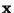linearly so that we obtain a new vector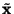which is white, i.e. its components are uncorrelated and their variances equal unity. In other words, the covariance matrix ofequals the identity matrix:(30)

The whitening transformation is always possible. One popular method for whitening is to use the eigen-value decomposition (EVD) of the covariance matrix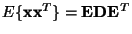, where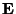is the orthogonal matrix of eigenvectors of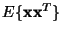and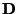is the diagonal matrix of its eigenvalues,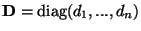. Note thatcan be estimated in a standard way from the available sample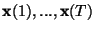. Whitening can now be done by(31)

where the matrixis computed by a simple component-wise operation as. It is easy to check that now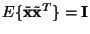.

Whitening transforms the mixing matrix into a new one,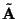. We have from (4) and (34):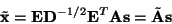(32)

The utility of whitening resides in the fact that the new mixing matrixis orthogonal. This can be seen from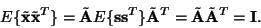(33)

Here we see that whitening reduces the number of parameters to be estimated. Instead of having to estimate the n2 parameters that are the elements of the original matrix, we only need to estimate the new, orthogonal mixing matrix. An orthogonal matrix contains n(n-1)/2degrees of freedom. For example, in two dimensions, an orthogonal transformation is determined by a single angle parameter. In larger dimensions, an orthogonal matrix contains only about half of the number of parameters of an arbitrary matrix. Thus one can say that whitening solves half of the problem of ICA. Because whitening is a very simple and standard procedure, much simpler than any ICA algorithms, it is a good idea to reduce the complexity of the problem this way.

It may also be quite useful to reduce the dimension of the data at the same time as we do the whitening. Then we look at the eigenvalues dj ofand discard those that are too small, as is often done in the statistical technique of principal component analysis. This has often the effect of reducing noise. Moreover, dimension reduction prevents overlearning, which can sometimes be observed in ICA .

A graphical illustration of the effect of whitening can be seen in Figure 10, in which the data in Figure 6 has been whitened. The square defining the distribution is now clearly a rotated version of the original square in Figure 5. All that is left is the estimation of a single angle that gives the rotation.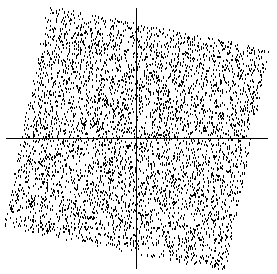In the rest of this tutorial, we assume that the data has been preprocessed by centering and whitening. For simplicity of notation, we denote the preprocessed data just by, and the transformed mixing matrix by, omitting the tildes.Next: Further preprocessing Up: Preprocessing for ICA Previous: Centering
Aapo Hyvarinen
2000-04-19